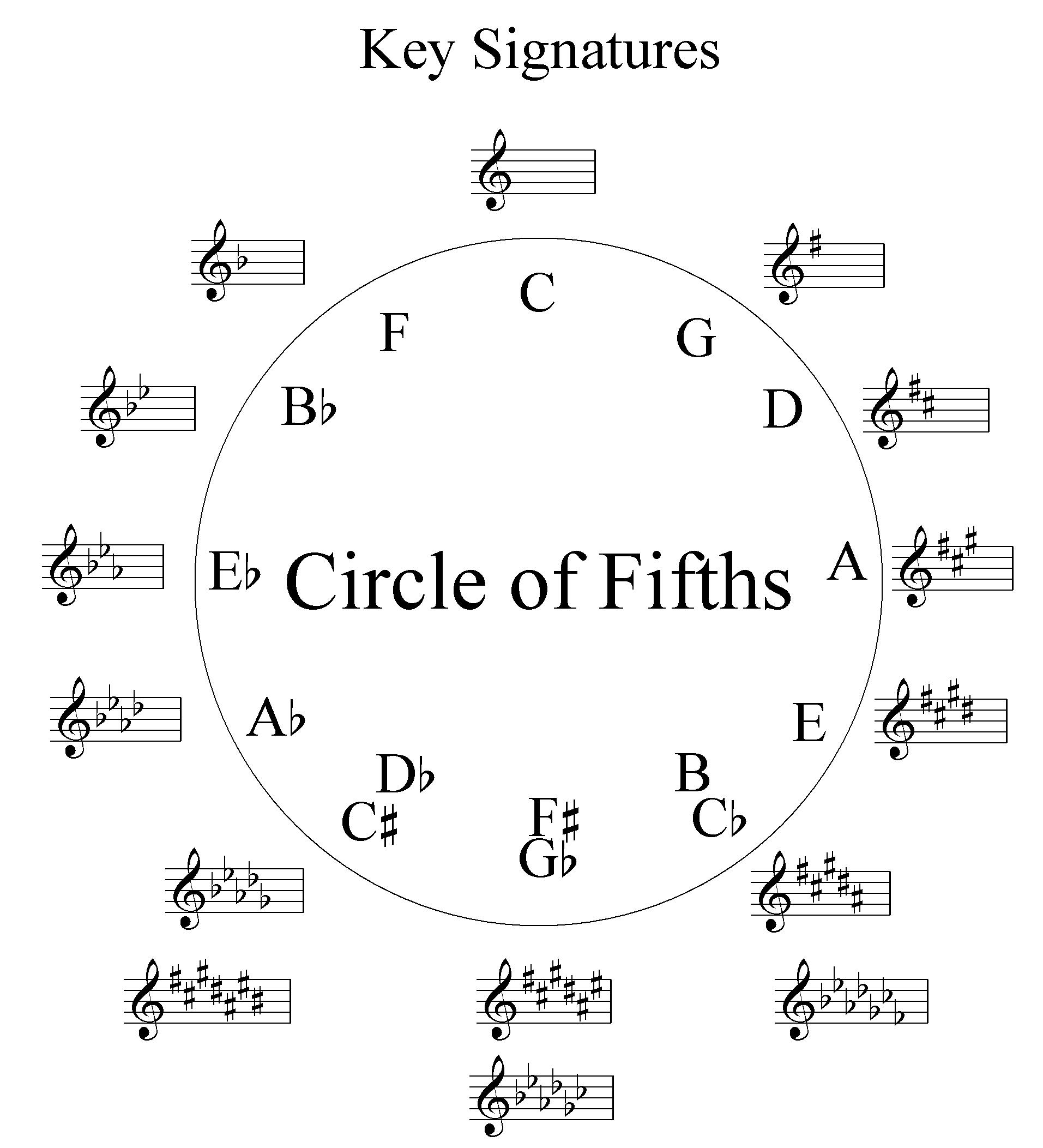# How the circle of fifths is described in my understanding

well, indeed it’s easier for us to understand the circle of fifth than the circle of fourths in guitar learning. There are two reason for this. first, we can always find the fifth note on the guitar fret board, simply by pick the string right above the root note (for the root note on the 3th, 4th, 5th string and the 1st string, the fifth note is right above it; for the 2nd string, the fifth note is half-note-down above).  it’s visually known by our eyes and our fingers can touch it right away.the second reason and the real reason is that the circle of fifth is obtained from the notes order linearly. there is something that we called tetrachord. the wikipedia definition:

a tetrachord is a series of three smaller intervals that span the interval of a perfect fourth, a 4:3 frequency proportion.

the circle of fifth is obtained from diatonic tetrachord (this is sh!t, there are too many difficult words for beginner guitarist like me to understand!). I’ll try to explain what is in my mind and let pictures redefine all these.

let’s divide an octave into 2 parts,
1 2 3 4 5 6 7 into 1 2 3 4 and 5 6 7 1
put them on a paper and write down the note intervals

now we have separated and identical intervals, 1 2 3 4 = w-w-h and 5 6 7 1= w-w-h

put the alphabet notes, then we have C D E F and G A B C plus the intervals.
the rest is easy. pay attention to the fifth note and observe how each fifth note is transferred onto the first(root) note. there you can see the number of sharped notes in each transferred note. here is the complete picture.

from that picture above, we can make a circle of notes called ” the circle of fifths”
here i share picture of it from wikipedia